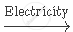Share

# Complete and Balance the Following Chemical Equations: Naci (Aq) + H_2o (L)  - Science

Course
ConceptChemicals from Common Salt Introduction

#### Question

Complete and balance the following chemical equations:

NaCI (aq) + H_2O (l)#### Solution

2NaCl (aq) + 2H_2O(l)2NaOH (aq) + Cl_2 (g) + H_2 (g)

Is there an error in this question or solution?

#### Video TutorialsVIEW ALL 

Solution Complete and Balance the Following Chemical Equations: Naci (Aq) + H_2o (L)  Concept: Chemicals from Common Salt Introduction.
S